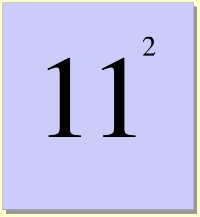Exponents 3
Set 3 (10 Questions)
Determine the prime factors of all numbers through 50 and write the numbers as the product of their prime factors by using exponents to show multiples of a factor (e.g., 24 = 2 x 2 x 2 x 3 = two cubed times three)
From Mr. Anker TestsExponents Help Slide 1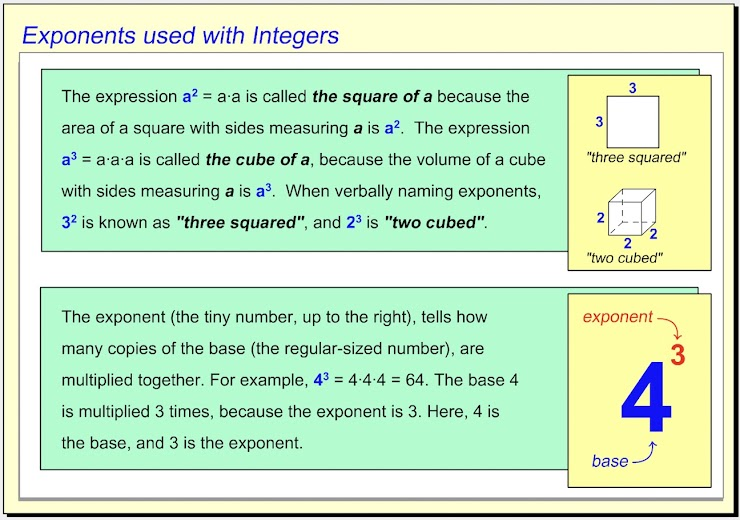Exponents Help Slide 2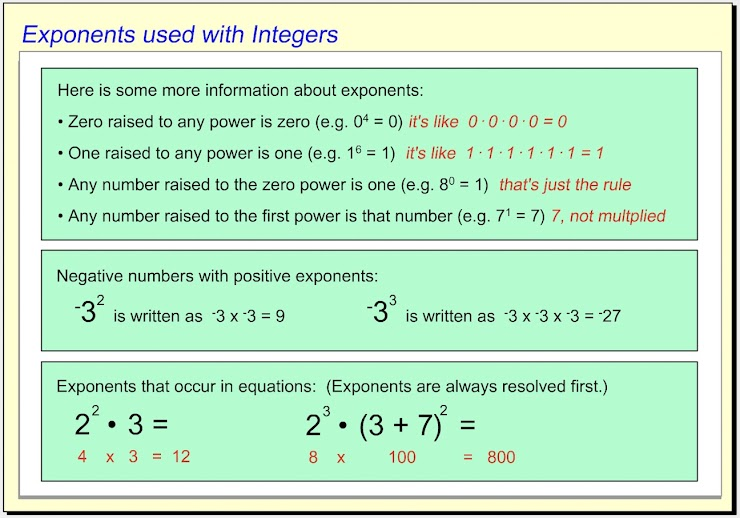Exponents Help Slide 3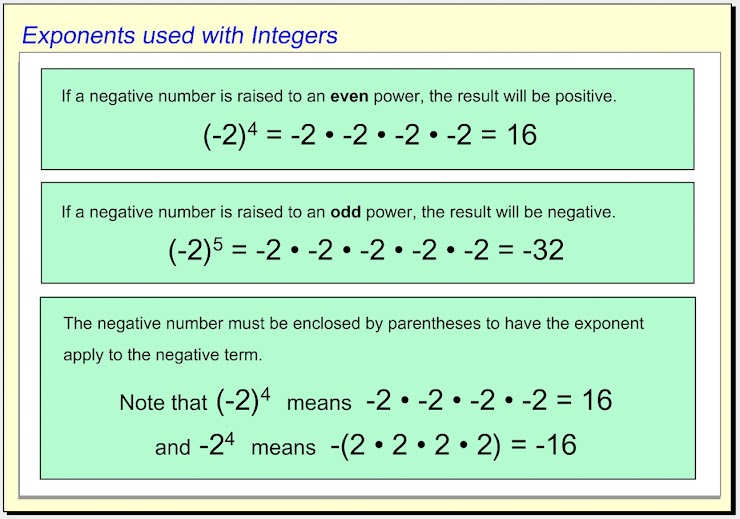1. Which of the choices below is equal to the integer with the exponent shown? *
1 point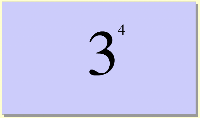2. Use the dropdown menu to make this statement true: "Three squared plus four squared is __________ two squared plus three cubed." *
1 point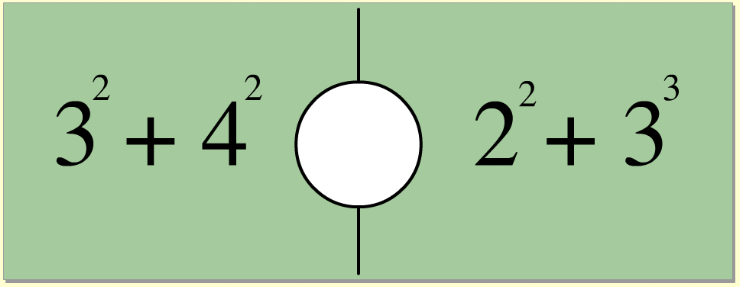3. Which of the choices below is equal to the integer with the exponent shown? *
1 point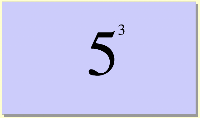4. Use the dropdown menu to make this statement true: "Five squared plus five squared is __________ five cubed." *
1 point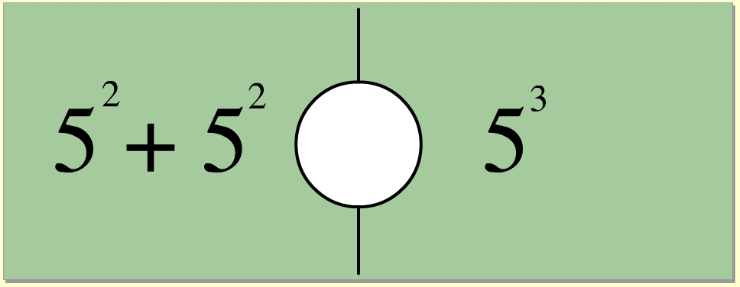5. Which of the choices below is equal to the integer with the exponent shown? *
1 point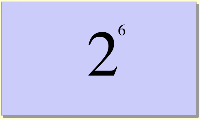6. Use the dropdown menu to make this statement true: "Five cubed minus five squared is ___________ ten squared." *
1 point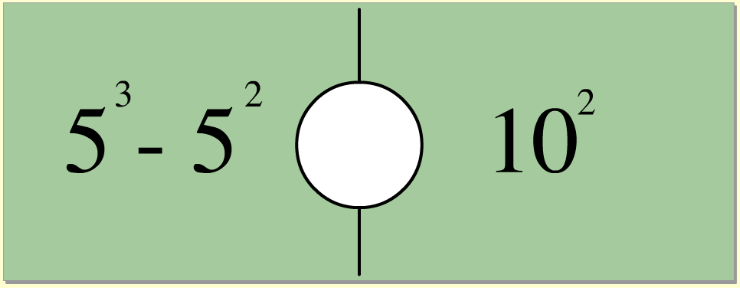7. On the line below, enter the equal value as a whole number. *
1 point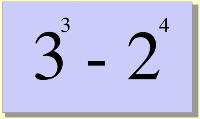8. Use the dropdown menu to make this statement true: "Two cubed times three squared is ___________ five squared times three." *
1 point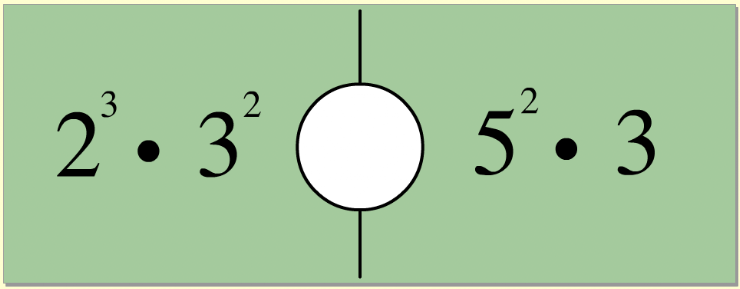9. Which of the choices below is equal to the integer with the exponent shown? *
1 point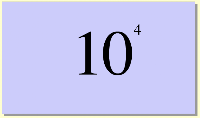10. On the line below, enter the equal value as a whole number. *
1 point